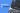FizzBuzz In Python

Recently I started looking for a new role, a new developer role. During my interviews, I have come across a number of questions and coding challenges. For each of these challenges, I take my time, create the code. I then come home and revisit that challenge to write a better solution.

In this new solution I create it in a few different languages, just to challenge myself, but to also provide my solution to anyone who maybe looking for the solution the this quiz question.

For this challenge I take one of the common coding quizzes I've seen asked during an interview is the FizzBuzz code question.

FizzBuzz

What is FizzBuzz? Simply put, its a challenge that will print one of 3 words when a number is divisible by a given number, and if not divisible it will output the number.

So, it seems common to give the following parameters:

1. Number range is 1 to 100
2. If the number is divisible by 3 then output Fizz
3. If the number is divisible by 5 then output Buzz
4. If the number is divisible by 3 and 5 then output Fizzbuzz
5. If the number is not divisible by the above values, output the value

FizzBuzz in Python

Given the above parameters, here is my solution for this FizzBuzz challenge written in Python

def fizz_buzz(count):
found = False

for current_value in range(1, count+1):
output_string = ""

if current_value % 3 == 0:
output_string = "Fizz"
found = True

if current_value % 5 == 0:
output_string += "Buzz"
found = True

if found == False:
output_string = current_value

print(output_string)
found = False

fizz_buzz(100)